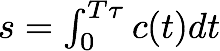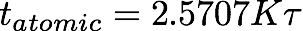Monday, October 27, 2008

Setterfield G

Here I'll continue my examination of Setterfield's new publication, “Reviewing A Plasma Universe With Zero Point Energy”, in the Journal of Vectorial Relativity 3, 1-29 (2008).

Fails to compare his model to data
In the last draft of the “Issues on Barry Setterfield's Claims of a Recently Decaying Speed of Light, 2nd Edition”, available here, I examined one of the latest functions Setterfield claimed represented the speed of light with cosmic (dynamical) age, which corresponded to his young-universe 'interpretation'. For bookkeeping purposes, I designated all the different functions from Setterfield by letters. I had labeled one of the latest functions Setterfield E.﻿
where T ranges from [0...1] and represents the time relative to the total age of the Universe. T=1 corresponds to the time of creation and gives the speed of light as infinite. But T=0 corresponds to the present day, and gives the nonsensical result that the speed of light equals ZERO!

T is chosen to be dimensionless, but it would be useful to clarify it as scaled relative to the (young) dynamical agewhere t is measured from the present time into the past and the greek letter τ is the total age of the universe.

Setterfield must have finally realized that blunder, since in his latest publication he has changed his function toor, defining a constant of proportionality,where c0 is the modern value for the speed of light, and we define the Greek letter ζ to be the dimensionless speed of light, chosen so that the value today is equal to unity or 1. I'll call this function 'Setterfield G'. (I had found an older function which I labeled 'Setterfield F' so that letter was already spoken for. Setterfield F appears on the graphs on the previous article.).

To compute the apparent age of the universe in Setterfield's new model, we must integrate the light travel time from the creation of the universe, T, to today, T=0. (Setterfield choses a mathematically reversed sense for time, increasing into the past, which creates a sign flip on our math.)orTo get an apparent travel time assuming a modern speed of light, divide both sides by c0 to getwhere K' is K τ and corresponds to Setterfield's equation 33. If we compute this for T=1 corresponding to the creation time, this gives the apparent age of the universe, in the atomic time scale:orLet's plug in some rough numbers using Setterfield's own prescription for computing the constant. If the (young) dynamical age of the universe, τ, is 7000 years in Setterfield's model, and the atomic age is 14 billion years, then K = 7.78e5.

But wait, K must be the exact same K value used for the speed of light earlier! If we use it in that equation, it tells us that the speed of light today (T=0) must be 778,000 times the current speed of light!

To get the correct value for the speed of light today, we must set K=1. But if we install that in the equation above, then we get the apparent age of Setterfield's universe is only about 18,000 years!

Setterfield's model is easily demonstrated to be mathematically and physically inconsistent!

This is a basic error that a high school physics or calculus student shouldn't make.

Did anyone do even a basic check of the math in this paper? Is such carelessness representative of the referee process at JVR?